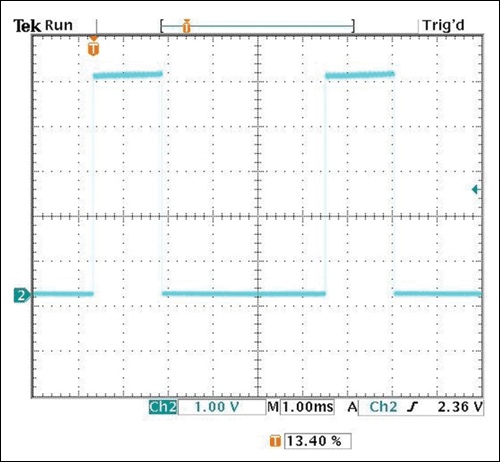# Simple Solutions for a Single-Device Pulse-Width Modulation (PWM) Waveform Generator

### 要約

Pulse-width modulation (PWM) generators are integrated in nearly every switching power device. The methods of implementing integrated PWM generators are well-known. This application note is prompted by a customer who asked for a single-device standalone analog PWM waveform generator.

A similar version of this article appears on EDN, September 25, 2013.

Pulse-width modulation (PWM) generators are integrated in nearly every switching power device. This article shows two methods for implementing a stand-alone analog PWM waveform generator. These designs can also be modified to make a dual-device PWM generator.

There are two ways to implement a single-device PWM waveform generator. One method uses an ICM7555 timer, while the other uses a MAX998 low-power comparator. We will look at each.

### Method 1: Use a Low-Power Timer as a PWM Generator

In this method an ICM7555 timer is configured as in Figure 1.In Figure 1 the pulse width of the output at Pin 3 is modulated by the control voltage (VCONTROL) applied at Pin 5. Lab tests were done on the design with the power supply set at 5V. Figures 2 through 5 show the PWM output at three different control voltages, 1V, 2V, and 4V. C1 is charged to VCONTROL by the supply voltage (VSUPPLY) and discharged from VCONTROL/2 to ground. When no external control voltage is applied, VCONTROL is at 2/3 of VSUPPLY.The data illustrate how the control voltage applied at Pin 5 changes the threshold voltage of the two internal comparators. Without the applied control voltage (Figure 4), the device sets the charging and discharging of C1 at 1/3 and 2/3 of the supply voltage. This is equidistant from the supply voltage and ground, thus effecting a 50% duty cycle. The different control voltages change the charging time for C1 to reach VCONTROL and the discharging time for C1 to discharge to VCONTROL/2. This process alters the pulse width of the output waveform.

The charging time is expressed as:

-t/RC = ln [1 – (VCONTROL/(2VSUPPLY - VCONTROL))]

The discharging time is expressed as:

-t/RC = ln 0.5
where R = R1 and C = C1.

### Method 2: A PWM Generator with Comparator

In this method a MAX998 comparator is configured as in Figure 6.The pulse width of the output is modulated by the control voltage applied at R1. Lab tests were done with the power supply set at 5V. Figures 7 through 9 show the PWM output of three different control voltages, 1V, 2V, and 3V.The control voltage applied to the MAX998 sets the threshold voltages at which charging and discharging occur. The upper threshold voltage is (VSUPPLY

VCONTROL)/2 + VCONTROL and the lower threshold voltage is VCONTROL/2.

The charging time is expressed as:

-t/RC = ln [1 – (VSUPPLY/(2 × VSUPPLY) - VCONTROL))]

The discharging time is expressed as:

-t/RC = ln [1 - (VCONTROL/(VSUPPLY + VCONTROL)
where R = R1 and C = C1.

### Modifications for a Dual-Device PWM Generator

It is important to note that the control voltage also changes the frequency in both circuit methods. Thus, an additional comparator to the circuits of Method 1 and Method 2 transforms each into a fixed-frequency, dual-device PWM generator.

For Method 1, feed the sawtooth signal at Pin 6 into an input of the second comparator. A voltage applied at the second comparator’s input sets the duty cycle of the fixed-frequency output. Similarly for Method 2, feed the sawtooth signal at the MAX998’s negative input into the input of the second comparator. A voltage applied at the second comparator’s input sets the duty cycle of the fixed-frequency output.